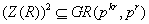• ### On the Zero Divisor Graphs of Finite Rings in Which the Product of Any Two Zero Divisors Lies in the Coefficient Subring

Abstract: Let r be a positive integer and 2≤k∈Z. Let GR(pkr, pk) be a Galois ring of order pkr and characteristic pk. Consider, R = GR(pkr, pk) ⊕U where U is a finitely generated GR(pkr, pk) module. If Z(R) is the set of zero divisors in R satisfying the conditionthen it is well known that R is a completely primary finite ring and the structure of its group of units has been studied before. In this paper, we study the structure of its zero divisors via the zero divisor graphs.